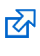# That Blue Square Thing

## AQA Computer Science GCSE

May 2019: this area of the site is being built just now. I’m making progress but there are areas where there is no content yet. That will get added over the next 6 months or so.

### Data Representation - Hexadecimal Numbers

Hexadecimal numbers use base 16 - so you can use one digit to county up to 15. The letters A to F are added on after 9, so A = 10, B = 11 and so on until F = 15. That gives you 16 possible values - including the 0.

Hexadecimal isn't used by computers, but it is an easy thing for humans to use when we're trying to talk in binary. And it converts really easily.

You need to be able convert hexadecimal to and from 8-bit binary numbers and to and from decimal numbers. Just remember that A = 10!Hexadecimal basics - notes on how it all works. The list on slide three is super important to know.The 16 times table - and how not to panic when doing the maths. Slides used in class.Hexadecimal questions - some hex to binary conversion questions

One really important thing to remember is that the hexadecimal number FF = 255 - which is the same as the 8 bit binary number 11111111. So, 255 is the largest number which can be represented using 8 bit binary (a byte) and a 2 digit hexadecimal number.

Just remember that A = 10 and F = 15. It's really easy to get into thinking that A is 11 and F is 16. It's not! Get this right and hexadecimal is a piece of cake.

#### Conversions using Hexadecimal

You need to be able to convert from:

• decimal to hexadecimal
• hexadecimal to decimal
• hexadecimal to binary (8 bit binary only)
• binary to hexadecimal (8 bit binary only)

I will write up the methods for this properly at some point (perhaps), but here are some photos taken in class which summarise the methods:

Decimal to Hex/Hex to Decimal

Binary to Hex/Hex to Binary

It's interesting to note that it's generally much easier to convert Hex to Binary. It's only when decimal gets involved that things become trickier.

#### Revision

There's likely to be one or two marks on hexadecimal on the exam.

The BBC Bitesize website has a section on hexadecimalthat you might want to look at. Well worth a look.